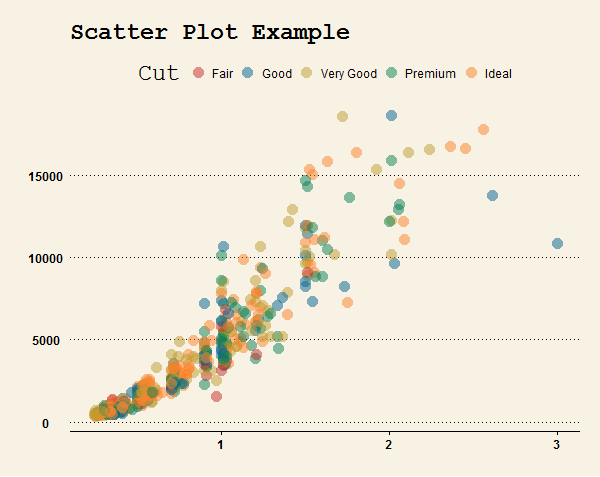Plot.ly is a great option to create and share beautiful interactive plots in R. Its R ggplot2 integration is an awesome feature and here is some basic steps that you can try and play with. All you have to do is to create an account and follow the installation steps. Explore The API libraries if you want to use Plot.ly in other languages such as Python.

Getting Started

For more detailed installation and initialization steps, visit plot.ly: getting started.

# installation
install.packages("devtools")
library("devtools")
install_github("ropensci/plotly")
library(plotly)

# authentication (only have to do this once)
# API key can be found in the settings page

Basic Steps

We use the diamonds data in ggplot2 package as an example. First let’s make a scatter plot of carat vs. price for each level of cut.

# initialize a plotly object
py <- plotly()
# make a scatter plot with ggplot2
sampledat <- diamonds[sample(nrow(diamonds), 500),]
p <- ggplot(data = sampledat, aes(carat, price, colour = cut)) + geom_point(size = 5, alpha = 0.5) +
labs(title = "Scatter Plot Example", x = "Carat", y = "Price", colour = "Cut")

# parsse the ggplot to plotly object and generate the interactive plot online
out <- py$ggplotly(p, kwargs = list(filename = "PlotlyScatterExample", fileopt = "overwrite")) # URL for the plot url <- out$response$url After publishing, you can use the Edit graph button to edit your plot. Usually this is a necessary step since the published plot loses some of the features in ggplot. For example, I had to manually set the point size and exponents labels to make the plot look similar to the original ggplot. Change Themes See the Github page of ggthemes for the full list of available themes, color palettes and examples. # install ggthemes package from CRAN install.packages('ggthemes', dependencies = TRUE) # OR install the development version from github library("devtools") install_github("ggthemes", "jrnold") ##### library(ggthemes) p1 <- p + theme_wsj() + scale_colour_wsj("colors6") py1 <- plotly() out1 <- py1$ggplotly(p1, kwargs = list(filename = "PlotlyThemeExample", fileopt = "overwrite"))
url1 <- out1$response$url

The generated Plot.ly plot is somewhat different from (and in my opinion, not as good-looking as) the original ggplot. Still, it is nice to have the option to change the color palettes and I am looking forward to the future updates on the theme features.

Comparison: the original ggplot with wsj theme.Embedding the Plots

It is very easy to embed the plots in HTML. See the tutorial for details.